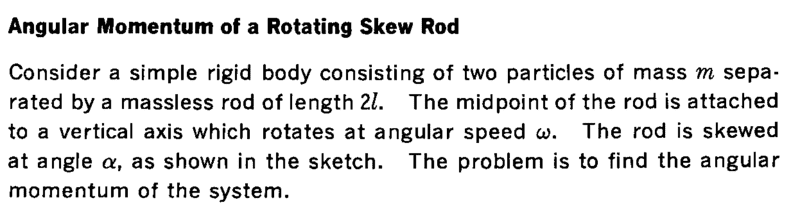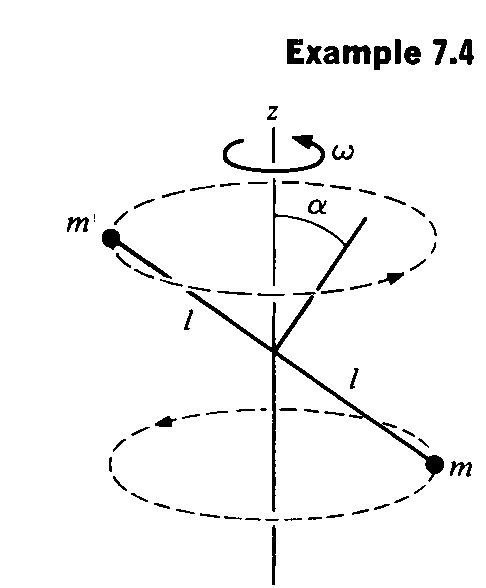# Angular momentum of a rotating skew rod

• Pushoam

## Homework Statement## The Attempt at a Solution

The angular momentum of the upper particle is given as
## \vec L_u = m \vec r \times ( \vec \omega \times \vec r )= m[ \vec \omega r^2 - \vec r ( \vec r . \vec \omega )] = m[ r^2 \vec \omega - \omega r \cos \alpha \vec r ]##
The angular momentum of the lower particle is same to that of the upper particle.
Hence, ##\vec L = 2 \vec L_u ##
Is this correct so far?

## Homework Statement

View attachment 209494
View attachment 209495

## The Attempt at a Solution

The angular momentum of the upper particle is given as
## \vec L_u = m \vec r \times ( \vec \omega \times \vec r )= m[ \vec \omega r^2 - \vec r ( \vec r . \vec \omega )] = m[ r^2 \vec \omega - \omega r \cos \alpha \vec r ]##
The angular momentum of the lower particle is same to that of the upper particle.
Hence, ##\vec L = 2 \vec L_u ##
Is this correct so far?
Check your expansion of ##\vec r . \vec \omega ##.

Check your expansion of ⃗r.⃗ωr→.ω→\vec r . \vec \omega .
After correction:
##
\vec L_u = m \vec r \times ( \vec \omega \times \vec r )= m[ \vec \omega r^2 - \vec r ( \vec r . \vec \omega )] = m[ r^2 \vec \omega - \omega r \sin \alpha \vec r ]##
Is this correct?

After correction:
##
\vec L_u = m \vec r \times ( \vec \omega \times \vec r )= m[ \vec \omega r^2 - \vec r ( \vec r . \vec \omega )] = m[ r^2 \vec \omega - \omega r \sin \alpha \vec r ]##
Is this correct?
Yes.

Yes.
But the angular momentum should be perpendicular to ## \vec r ##. Here, I am getting non-zero component in the direction of ##\hat r##.

But the angular momentum should be perpendicular to ## \vec r ##. Here, I am getting non-zero component in the direction of ##\hat r##.
The other term, ##\vec\omega##, also has a component in the direction of ##\vec r##. The two may cancel.

The other term##\vec\omega##, also has a component in the direction of##\vec r##. The two may cancel.
Yes, it gets canceled.
Thanks for the guidance, thanks a lot.